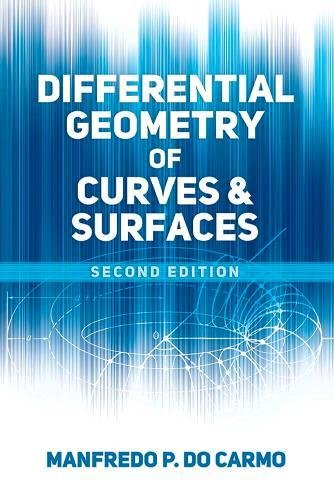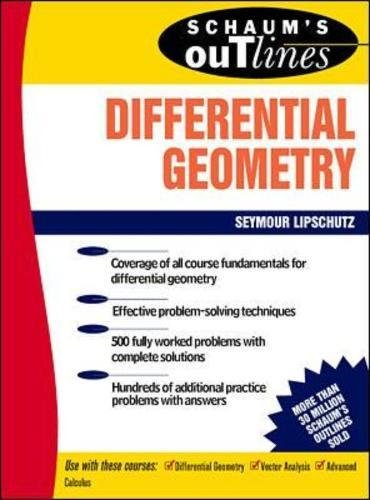# Best Differential Geometry Books | Enriching Mathematics Knowledge

Differential Geometry is a branch of mathematics that uses the techniques of differential calculus, integral calculus, linear algebra, multilinear algebra, curves, surfaces, and manifold and is closely related to the differential topology. Here you will get some of the best differential geometry books.

## 1. Differential Geometry (Dover Books on Mathematics)

Differential Geometry is a very informative book which covers many important topics including nature and purpose of differential geometry, a concept of mapping, coordinates in Euclidean space, vectors in Euclidean space, basic rules of vector calculus in Euclidean space, tangent and normal plane, osculating plane, involutes, and evolutes, Bertrand curves etc.

This book contains the following eight chapters
• Preliminaries
• Theory of curves
• The concept of a surface, First fundamental form, foundations of the tensor, calculus
• Second fundamental form, Gaussian and mean curvature of a surface
• Geodesic curvature and Geodesics
• Mappings
• Absolute differential and parallel displacement
• Special surfaces.

## 2. Differential Geometry of Curves and Surfaces: Revised and Updated Second Edition (Dover Books on Mathematics)Author: Manfredo P. do Carmo
Published at: 14/12/2016
ISBN: 0486806995
Differential Geometry of Curves and Surfaces answers all of the problems with examples and exercises that you face in differential geometry. This book is suitable for undergraduates and graduate students of mathematics and introduces the differential geometry of curves and surfaces in both local and global aspects.

Key topics are

1. Curves
2. Regular surfaces
3. The geometry of the Gauss map
4. The intrinsic geometry of surfaces
5. Global differential geometry

You'll also get an idea about linear algebra, calculus of several variables in a corrected, revised, and updated way.

## 3. Schaum's Outline of Differential Geometry (Schaum's)Author: Martin M. Lipschutz
Published at: 01/06/1969
ISBN: 0070379858

Schaum's Outline of Differential Geometry offers practice problems with full explanations, coverage of the most up-to-date developments in your course field, in-depth review of practices and applications that reinforce your knowledge. Schaum's is the key to faster learning and higher grades in every subject that provides you hundreds of examples, solved problems, and practice exercises to test your skills.

Important coverage are

• Intrinsic geometry
• Tensor analysis
• Theory of surfaces
• First and second fundamental forms
• Concept of curve
• Vector functions of a vector variable
• Elementary topology in Euclidean spaces
• Theory of curves
• Curvature and torsion
• Concept of curve
• Vector functions of a real variable
• Vectors.

## 4. A Comprehensive Introduction to Differential Geometry, Vol. 1, 3rd EditionAuthor: Michael Spivak,Michael Spivak
Published at: 01/01/1999
ISBN: 0914098705

Michael Spivak is the master of Differential Geometry. He provides all the basic definitions with lots of examples of each and every topic. This is the first volume of the 3rd edition in a five-volume series on differential geometry. This 5 volume set is classic and overall the best and most thorough treatment of differential geometry. You'll get a clear and concise idea about every topic without the aid of an instructor.

Key coverage are

• Manifolds
• Differential structures
• The tangent bundle
• Tensors
• Vector fields and differential equations
• Integral manifolds
• Differential forms
• Integration
• Riemannian metrics
• Lie Groups
• Excursion in the realm of algebraic topology.

## 5. Elementary Differential Geometry, Revised 2nd Edition

Elementary Differential Geometry is appropriate for self-study as it contains numerous exercises with answers. This is a perfect guide to build your fundamental knowledge of the subject.

This book is well recommended for undergrads as well as for students who have completed the standard first courses in calculus and linear algebra. It also contains solutions for odd number problems.

The most important topics are

• Introduction
• Calculus on Euclidean space
• Frame fields
• Euclidean geometry
• Calculus on a surface
• Shape operators
• The geometry of surfaces in R3
• Riemannian geometry
• Global structures of surfaces.

## 6. Lectures on Classical Differential Geometry: Second Edition (Dover Books on Mathematics)

Dirk J. Struik explains the equations in a clean and sparse way. The notation is also straightforward and always clear, unambiguous and economical. This guide is perfect for self-study.

This book offers you

• An excellent brief introduction to the classical theory of differential geometry
• Visual understanding of geometry
• Appendix with a sketch of the application of Cartan's method of Pfaffians to curve and surface theory.
It is for

## 7. Differential Geometry of Curves and Surfaces (Undergraduate Texts in Mathematics)

Differential Geometry of Curves and Surfaces is a great book for undergraduates that include plenty of examples and intermediate steps within proofs while providing an invitation to more excursive applications and advanced topics.

Color is used to highlight important topics like Green-boxed definitions and purple-boxed theorems help to visually organize the mathematical content.

Key topics are

• Introduction
• Curves
• Surfaces
• The curvature of a surface
• Geodesics
• The Gauss-Bonnet theorem.

## 8. Introduction to Differential Geometry of Space Curves and Surfaces: Differential Geometry of Curves and Surfaces

This book is a must-have for the math student. The explanation and illustration made this book a very effective one. This guide reduces the need for external resources.

Introduction to Differential Geometry of Space Curves and Surfaces can be used as

• Part of a course on tensor calculus as well as a textbook
• A reference for an intermediate-level course on the differential geometry of curves and surfaces.

This book provides

• An index, extensive sets of exercises and many cross-references
• A considerable number of 2D and 3D graphics illustrations
• Help to visualize the ideas and understand the abstract concepts
• Offered materials of differential geometry to make the book fairly self-contained.

## 9. Differential Geometry: Connections, Curvature, and Characteristic Classes (Graduate Texts in Mathematics)

Differential Geometry is a graduate-level book for both the mathematics and physics students. There include Appendix A recalls the basics of manifold theory, sections on algebraic constructions such as the tensor product and the exterior power.

This guide provides six chapters

• Curvature and vector fields
• Curvature and differential forms
• Geodesics
• Tools from algebra and topology
• Vector bundles and characteristics classes
• Principal bundles and characteristics classes

Differential geometry is useful in

• Topology
• Several complex variables
• Algebraic geometry
• Complex manifolds, and
• Dynamical systems.

Thanks for reading this post. If you have any opinion don't hesitate to comment here. Also please subscribe our newsletter to get more updates.